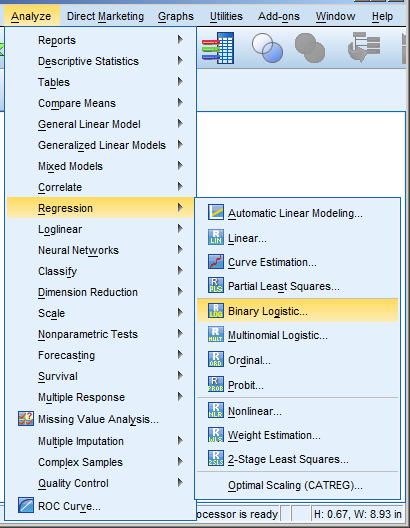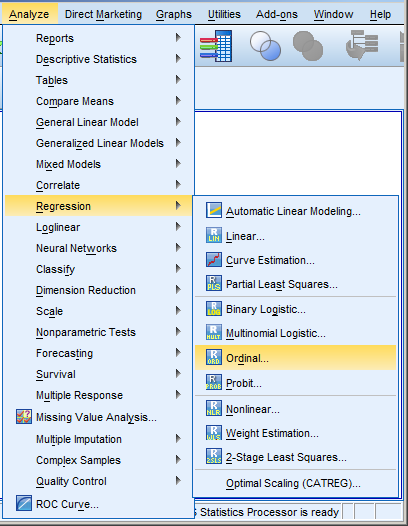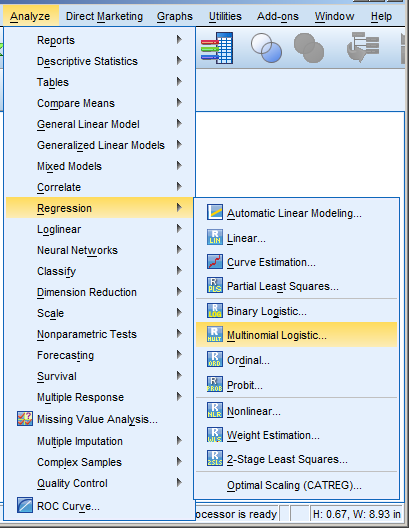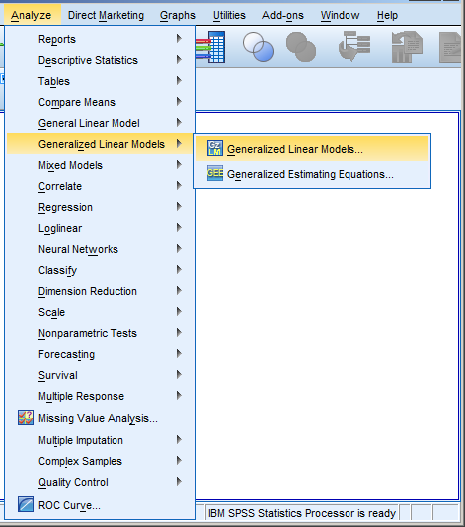# SPSS Procedures for Logistic Regression

Need to run a logistic regression in SPSS? Turns out, SPSS has a number of procedures for running different types of logistic regression.

Some types of logistic regression can be run in more than one procedure.  For some unknown reason, some procedures produce output others don’t.  So it’s helpful to be able to use more than one.

### Logistic RegressionLogistic Regression can be used only for binary dependent variables.  Invoke it using the menu choices at right or through the LOGISTIC REGRESSION syntax command.

The dependent variable must have only two values.  If you specify a variable with more than two, you’ll get an error.

One big advantage of this procedure is it allows you to build successive models by entering a group of predictors at a time.

LOGISTIC REGRESSION VARIABLES BinaryDV
/METHOD=ENTER Factor Covariate1
/METHOD=ENTER Covariate2 Covariate3
/CONTRAST (Factor)=Indicator.

So for example, using this syntax, I’m actually simultaneously running two models, one with only two independent variables, (cleverly) named Factor and Covariate1.

The second model has four independent variables: FactorCovariate1, Covariate2, and Covariate3.

This is called “Hierarchical Regression” (not to be confused with Hierarchical Linear Models or HLM). It allows an easy comparison of how model fit and coefficients change as you add predictors.

The Logistic Regression command is invoked through the  menus under Regression–>Binary Logistic, as seen above.  (But don’t forget to Paste your syntax, so you have a record of what you did)!

### PLUM

PLUM stands for Polytomous Universal Model.  That’s a mouthful, but just comes down to models for categorical outcomes with more than two ordered categories.

If your outcome categories are not ordered, don’t use PLUM.  You cannot tell it that categories have no order, and it will put them into a logical order.

PLUM OrdinalDV BY Factor WITH Covariate
/PRINT=FIT PARAMETER SUMMARY.Logistic Regression models are one type of generalized linear model.  PLUM can actually fit 5 types of generalized linear model for ordinal outcomes, including probit and complimentary log-log models.

The LINK=logit command specifies the logistic model.

Logistic regression models in PLUM are proportional odds models.  That means a few things. First,  it models the odds for each ordered category compared to all lower-ordered categories. Second, the odds ratio for each predictor is the same, regardless of whether you’re comparing category 4 to 3 and below or category 3 to 2 and below.

PLUM has a nice option to check whether this assumption is reasonable.  In many data sets it isn’t, so always check it.

PLUM is invoked through the  menus under Regression–>Ordinal, as seen above.

### NomReg

NomReg fits Multinomial Logistic Regression models for nominal outcomes.  That means outcomes with more than two unordered categories.

Unlike binary and ordered models, multinomial models cannot also be run in GenLin (see below).

NOMREG MultinomialDV (BASE=LAST ORDER=ASCENDING) BY Factor WITH Covariate
/MODEL=Factor Covariate
/INTERCEPT=INCLUDE
/PRINT=PARAMETER SUMMARY LRT CPS STEP MFI.Multinomial logistic regression models simultaneously run a series of binary models. Each one compares the odds of one outcome category to a reference category.

One nice feature in NomReg is you can specify any one of the outcome categories as the reference. Just use the BASE= option (or clicking the “Reference Category” button in the menus).

In NomReg you can specify predictors as categorical by placing them after the BY option (or in the Factor box in the menu dialog) or as continuous by placing them after the WITH option (or in the Covariates box in the menu dialog). This is identical to many other SPSS procedures, like PLUM, GLM, and Mixed.

This can save you a lot of time creating dummy variables for categorical predictors.

### GenLin

As mentioned above, logistic regression models are one type of generalized linear model.This means that you can use the GenLin procedure to run binary and ordinal logistic regression models.  It doesn’t however, run unordered multinomial models.

GenLin can run many more models that just logistic.  So it requires that you specify the outcome distribution as either binomial or multinomial (for which it will run an ordinal model) and a logit link function.

GENLIN BinaryDV (REFERENCE=LAST) BY Factor (ORDER=ASCENDING) WITH Covariate
/MODEL Factor Covariate Factor*Covariate INTERCEPT=YES
/PRINT CPS DESCRIPTIVES MODELINFO FIT SUMMARY SOLUTION.

If you could use Logistic or PLUM, why would you ever use GenLin?

GenLin has a few advantages in certain situations.  Here are three that I commonly use.

1. GenLin can run binary models in the Events/Trials format.  Logistic can’t.

2. GenLin prints EMMeans in both the original scale (ie. probabilities) and the transformed scale (log-odds).

This is a huge advantage if you have categorical predictors.  One or two categorical predictors are not hard to interpret using the regression coefficients. But if you have many, if they have many categories per predictor, or if you have interactions among them, the means are much easier to interpret.

3. GenLin can run repeated measures models using Generalized Estimating Equations.  Again, this is not just an advantage, but a necessity, if you have a repeated measures design.Binary, Ordinal, and Multinomial Logistic Regression for Categorical Outcomes
Get beyond the frustration of learning odds ratios, logit link functions, and proportional odds assumptions on your own. See the incredible usefulness of logistic regression and categorical data analysis in this one-hour training.

1.weldu says

interested guide for my thesis.Thank you!

2.Lindsay Lucas says

Hi Karen, do you have any tips for finding the predicted probabilities for a certain value of a continuous variable. It is so simple in R and SAS but I can’t find a way to do it in SPSS other than writing out the equation manually.

Thanks,
Lindsay

•Lindsay Lucas says

I am currently using GENLIN with the repeated statement.

3.Martin says

Just one question,

is it possible to use the /METHOD=ENTER-command to create a sequential regression for the NOMREG model, too?

•Jabessa Hatahu says
4.Dr Albano Mbyuzi says